# Transmission Line Model

Light, phase and group velocities

This page was recently amalgamated from material from propagation constant, phase velocity and characteristic impedance pages.

The generalized lumped-element model of a transmission line can be used to calculate characteristic impedance, phase velocity, and both parts of the propagation constant (phase and attenuation). The model uses an infinitesimally small section of a transmission line with four elements as shown below. Here the series resistance, series inductance, shunt conductance and shunt capacitance are all normalized per unit length (denoted by the "prime" notation).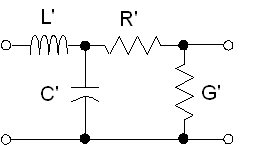Let's examine the relationships between phase constant, frequency, phase velocity and wavelength. Recall that there are 2π radians in a wavelength, therefore the relationship between phase constant and wavelength is simply: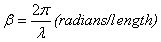Here's phase constant as function of frequency: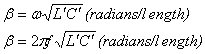Note that the phase constant is proportional to frequency. It also turns out that the expression SQRT(L'C') is the reciprocal of phase velocity of the transmission line. Here's a separate page on that topic! But for now, remember that it is always less than or equal to the speed of light in a vacuum, which is "approximately"2.99792458E+08 meters per second.

For completeness, here's some expressions for wavelength in terms of phase constant, or frequency: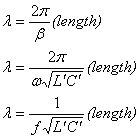The series impedance and shunt admittance of the structure are simply: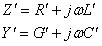The general form for the propagation constant starts out as this simple expression: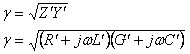### Propagation constant of lossless transmission line

If the transmission line is lossless, then R' and G' terms in the propagation constant equation are zero. For the lossless case the lumped model reduces to: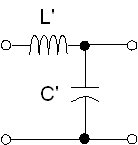If R' and G' terms in the propagation constant equation are zero, the attenuation constant is also zero. The general equation for propagation constant is neatly simplified: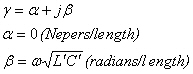In the case of a lossless transmission line, the propagation constant is purely imaginary, and is merely the phase constant times SQRT(-1):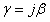### Propagation constant of low-loss transmission line

The propagation constant equation does not easily separate into real and imaginary parts for α and β in the case where R' and G' are non-zero terms. But significant approximations can be made for "low-loss" transmission lines. For these approximations to hold, these conditions must be met: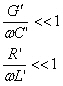What does low-loss mean here? Let's assume that the ratios in the above relations are held to 10%. We made a calculation for PTFE coax, 50 ohms, at 10 GHz. At the conditions described, R'=1500 ohms/meter, and G'=0.6 Siemens/meter. Both would result in losses of 130 dB/meter (or 0.13 dB/mm). This is a very lossy cable by lab standards. Although this is slightly oranges/apples comparison the condition is even lossier than what you might measure on a MMIC transmission line at X-band. Note that the condition scales with frequency, W-band signals can have ten times as much loss and still meet the condition. So the approximation holds for just about any transmission line, no worries!

Now on to the propagation constant equation. Approximations are made using the first two terms of a Taylor series expansion. We refer you to Pozar's excellent book if you want to study this. Here's the separated phase and attenuation constants.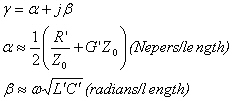Note that the phase constant is calculated exactly the same from way from capacitance and inductance per unit length, regardless if the transmission line is lossy or not.

You get a non-zero attenuation constant if either G' or R' in the transmission line model (above) are non-zero terms (when G' and R' are zero the transmission line is lossless and α=0). The approximation of the attenuation constant under these conditions is calculated as: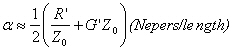In microwave engineering, we tend to separate the attenuation constant into different components. The mechanisms of series resistance and shunt conductance can be separated into two independent loss expressions: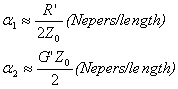The term alpha1 is actually the metal loss in a transmission line due to the skin depth effect. The term alpha2 can be further separated into loss due to dielectric loss tangent, and loss due to substrate conductivity.

We have a separate page on transmission lines loss that deals further with the topic of splitting up the attenuation constant, check it out!

### Velocity of light in a transmission line

phase velocity can mean something very different when we discuss waveguide.

Velocity of light can be derived from the inductance and capacitance per unit length of a transmission line. Under the normal (los-loss) conditions of: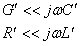The velocity of light in the transmission line is simply: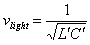For a TEM transmission line (coax, stripline) with air dielectric the velocity of light reduces to the constant "c" which is the velocity of light in a vacuum (2.997E8 maters/second).

### Transmission line characteristic impedance

The general expression that defines characteristic impedance is: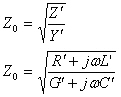Note that in its general form, characteristic impedance can be a complex number. Also note that it only becomes complex if either R' or G' are non-zero, which will give you a headache if you think about it too long. In practice we try to achieve nearly lossless transmission lines. For a low-loss transmission line, the following relationships will occur:Then for all practical purposes we can ignore the contributions of R' and G' from the equation and end up with a nice scalar quantity for characteristic impedance. For lossless (or near loss-less) transmission lines the characteristic impedance equation reduces to: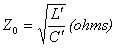What are L' and C' to the lay person? L' is the tendency of a transmission line to oppose a change in current, while C' is the tendency of a transmission line to oppose a change in voltage. Characteristic impedance is a measure of the balance between the two. How do we calculate L' and C'? that depends on what the transmission line is. For example our page on coax give the coax equations.

### Relationship of L' and C' to Z0 and VP

There are many situations where you need to know inductance per unit length and capacitance per unit length of a transmission line. Both can be calculated from the characteristic impedance and the propagation velocity of the wave in a transmission line. The key to solving these equations is that the propagation velocity of a transmission line is a very simple function of its capacitance and inductance per unit length: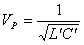Note than when you plug in inductance in Henries/meter and capacitance in Farads/meter, you are talking about one speedy wave, limited to be less than or equal to the velocity of light which is about 3x10E8 meters/second. From this equation and that for Z0 (above) you can arrive at the following for L' and C':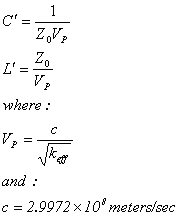Now the truth comes out... for a TEM transmission line such as stripline or coax, and for a given dielectric material (which would correspond to Keff in the above equations), the inductance and capacitance per unit length don't change when you scale the geometry up and down. So all semirigid, 50 ohm PTFE-filled cables (and PTFE-filled stripline!) will have 94.8 pF/meter (28.9 pF/foot) capacitance and 237 nH/meter (72.2 nH/foot) inductance! Let's state that as a Microwaves101 Rule of Thumb: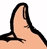For coax and stripline 50 ohm transmission lines that employ PTFE dielectric (or any dielectric material with dielectric constant=2), the inductance per foot of is approximately 70 nH, and the capacitance per foot is about 30 pF.

Author : Unknown Editor